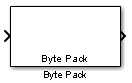# Byte Pack

Convert input signals to `uint8` vector

•Libraries:
Embedded Coder / Embedded Targets / Host Communication

## Description

The Byte Pack block receives input signals of one or more data types and converts the data to one `uint8` vector for output. Use block parameters to specify data types of the input signals and the alignment of the data in the vector that the block outputs. Because the UDP protocol transmits data in `uint8` format, you can use this block to reformat data for UDP transmission by connecting the output of this block to the input of a UDP Send block.

## Ports

### Input

expand all

Array of input signals of one or more data types.

Data Types: `single` | `double` | `int8` | `int16` | `int32` | `int64` | `uint8` | `uint16` | `uint32` | `uint64` | `Boolean` | `fixed point` | `enumerated` | `bus`

### Output

expand all

Converted block input, returned as a vector of `uint8` data.

Data Types: `uint8`

## Parameters

expand all

Specify the Simulink data types of input signals that the block receives in a cell array. In the cell array, specify data types in the order in which the block input port receives the signal data. For example, if the block receives data in the order `uint32`, `uint32`, `uint16`, `double`, `uint8`, `double`, and `single`, specify this cell array:

``` {'uint32','uint32','uint16','double','uint8','double','single'} ```

The block then provides the number of block inputs.

Specify how to align the data types of input data to form the `uint8` vector output in bytes. Alignment can occur on 1, 2, 4, or 8-byte boundaries. Based on the alignment value, each signal data value begins on multiples of the alignment value. The alignment algorithms for each element in the output vector begin on a byte boundary specified by the alignment value. Byte alignment sets the boundaries relative to the starting point of the vector.

To get the tightest packing without holes between data types in the various combinations of data types, select `1`.

You can have multiple data types of varying lengths. In such cases, a 2-byte alignment can produce 1–byte gaps between `uint8` or `int8` values and another data type. In the pack implementation, the block copies data to the output data buffer 1 byte at a time.

For example, assume that you specify this cell array for Input port data types (cell array):

``` {'uint32','uint32','uint16','double','uint8','double','single'} ```

Assume that you set Byte alignment to `2`. Each new value begins 2 bytes from the previous data boundary. When the signals are scalar values, the:

• First signal value in the vector starts at 0 bytes.

• Second signal value starts at 2 bytes.

• Third signal value starts at 4 bytes.

• Fourth signal value starts at 6 bytes.

• Fifth signal value starts at 8 bytes.

• Sixth signal value starts at 10 bytes

• Seventh signal value starts at 12 bytes.

The packing algorithm leaves a 1-byte gap between the `uint8` data value and the double value.

## Version History

Introduced in R2011a